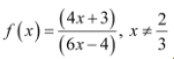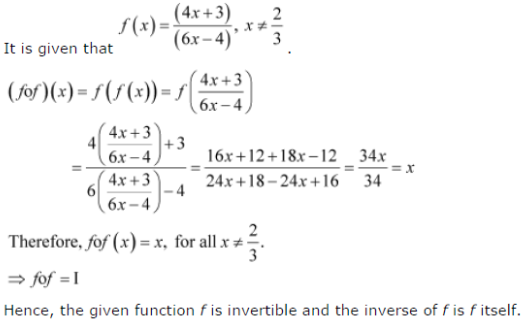# If f(x) = (4x+3) / (6x-4). show that fof (x) = x, for all x ≠ 2/3. What is the inverse of f?

If,
show that fof (x) = x, for all x ≠ 2/3. What is the inverse of f ?